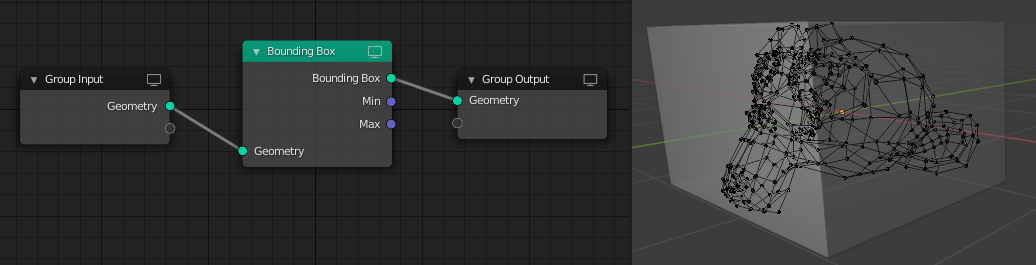# 边界框¶The Bounding Box node.

The Bounding Box node creates a cube with the minimum volume that encapsulates the geometry of the input. The node also can output the vector positions of the bounding dimensions.

## 输入¶

Standard geometry input.

## 输出¶

The resulting box or cube to encapsulate the input geometry.

The coordinates corresponding to the cubes -X, -Y, -Z position values, i.e. how far the box extends in each of the negative axes directions.

The coordinates corresponding to the cubes +X, +Y, +Z position values, i.e. how far the box extends in each of the positive axes directions.

## 例子¶Bounding Box node used to create a cube that encapsulates the monkey mesh geometry.